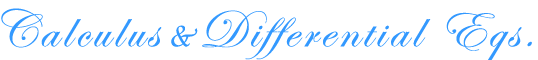The Center for
Mathematics and Quantitative Education at DartmouthAlgebra Art Biology, Medicine & Psychology Business & Economics Calculus & Differential Equations Computer Science Data Analysis & Statistics Discrete Math Engineering Financial Literacy Health Quantitative Literacy History & Geography K-12 Resources Literature & Writing Math & Music Physical Sciences Pre-Algebra Quantitative Literacy

Open Calculus

Open Calculus

Open Calculus is an exportable distance-learning/self-study environment for learning calculus. Embodied in this open source project is a calculus text, online homework problems, videotapes of worked examples, and more, which have been organized and linked together in a flexible fashion.

That's Calculus

That's Calculus
A video review of basic calculus concepts, including chemistry applications such as reaction times and radioactive decay.

by Edwin Gailits, Marcia Groszek and Dorothy Wallace

Order the series here, or individual discs on Amazon

Case Studies in Calculus

Case Studies in Calculus
A collection of worksheets promoting in depth exploration of selected applications of calculus, including "Torricelli's Law" and "Sleuthing Galileo."

by Don Kreider and Dwight Lahr

Calcsite

Calcsite
A website containing practice problems and tutorial videos.

By Dwight Lahr and Dorothy Wallace

Principles of Calculus Modeling

Principles of Calculus Modeling
A slim volume to accompany the case studies.

by Victor A. Stanionis, Hugh Berberich

Situated Complexity

Ordinary Differential Equation Machine

The Big Green Ordinary Differential Equation Machine
A Java applet that integrates systems of ordinary differential equations.

By B P Reid

The Bell That Rings Light

The Bell That Rings Light
A Primer in Quantum Mechanics and Chemical Bonding.

by Dorothy Wallace & Joseph J BelBruno

Makin' Waves

Makin' Waves
An introduction to Fourier series and the wave equation.

by Dorothy Wallace

Eyes on the Earth

Eyes on the Earth, I, II, III
Three videos exploring satellite imaging techniques from the point of view of an artist, a geologist, and a mathematician.

by Richard Birnie, Edwin Gailits, and Dorothy Wallace

An Introduction to Dynamical Systems And Mathematical Modelling